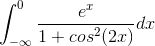# Integration help!

## Homework Statement

Calculate the integral:-

## The Attempt at a Solution

I tried some trig identities, like t=tan(x/2). The cos^2 smells like an arctan derivative but I can't seem to think of anything that could work...

You know cos^2(2x) is non-negative so it means 1+ cos^2(2x) is always positive
Use the fact that 1+cos^2(2x) is bounded to evaluate this integral .

Last edited:
Maybe write cos in exponents?

Ray Vickson
Homework Helper
Dearly Missed

## Homework Statement

Calculate the integral:-

## The Attempt at a Solution

I tried some trig identities, like t=tan(x/2). The cos^2 smells like an arctan derivative but I can't seem to think of anything that could work...

I am beginning to doubt there is a simple closed-form solution. However, one can reduce it to a finite integration that might be preferable to use if you want an accurate numerical value. Call the integral J, and note that we can re-write it as an integral over [0,∞):
$$J = \int_0^{\infty} f(x) \, dx, \:\: f(x) = \frac{e^{-x}}{1 + \cos^2(2x)}$$
Since ##\cos^2(2x)## is periodic with period ##\pi/2## we have
$$f\left( n \frac{\pi}{2} + t \right) = \alpha^n f(t), \; \alpha = e^{-\pi/2}$$
so
$$J = \sum_{n=0}^{\infty} \alpha^n J_0 = \frac{J_0}{1-\alpha}, \text{ where } J_0 = \int_0^{\pi/2} f(x) \, dx.$$
For numerical work it might be better to work with J_0 instead of the original J.